# Let G be a connected graph with m 2 vertices of odd degree. Prove that once is m/2. Let G be a connected graph with m 2 vertices of odd degree. Prove that once is m/2.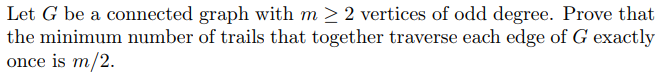Let G be a connected graph with m 2 vertices of odd degree. Prove that once is m/2.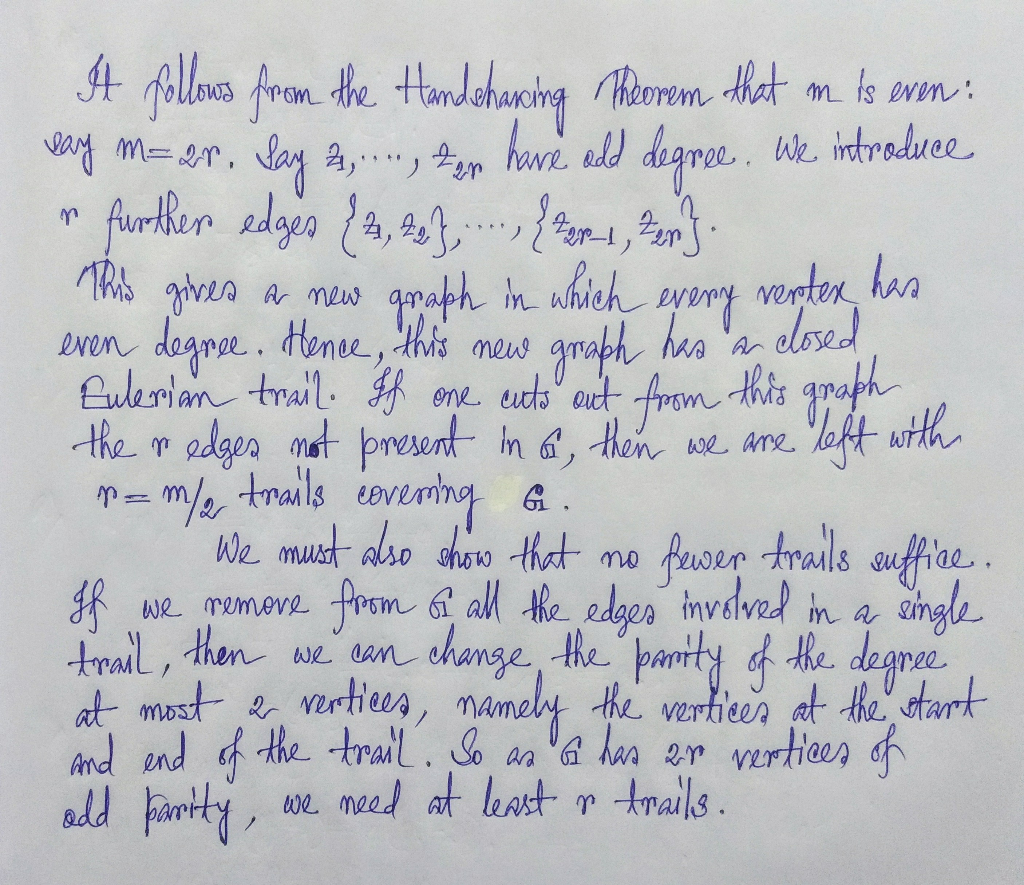##### Add Answer of: Let G be a connected graph with m 2 vertices of odd degree. Prove that once is m/2. Let G be a connected graph with m 2 vertices of odd degree. Prove that once is m/2.
Similar Homework Help Questions
• ### 49.12. Let G be a graph with n 2 2 vertices. a. Prove that if G has at least ("21) +1 edges, then...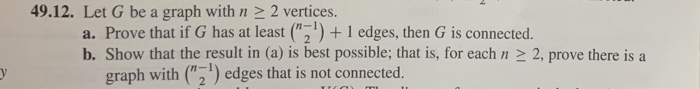49.12. Let G be a graph with n 2 2 vertices. a. Prove that if G has at least ("21) +1 edges, then G is connected. b. Show that the result in (a) is best possible; that is, for each n 2 2, prove there is a graph with ("2) edges that is not connected. 49.12. Let G be a graph with n 2 2 vertices. a. Prove that if G has at least ("21) +1 edges, then G is...

• ### (a) Let L be a minimum edge-cut in a connected graph G with at least two vertices. Prove that G −...

(a) Let L be a minimum edge-cut in a connected graph G with at least two vertices. Prove that G − L has exactly two components. (b) Let G an eulerian graph. Prove that λ(G) is even.

• ### Let G be a graph in which there is a cycle C odd length that has vertices on all of the other odd...

Let G be a graph in which there is a cycle C odd length that has vertices on all of the other odd cycles. Prove that the chromatic number of G is less than or equal to 5.

• ### Let G be a connected non-complete graph with order n 2 3 and diameter d. Prove that the connectivity κ(G) of G satisfies d-1 Let G be a connected non-complete graph with order n 2 3 and diameter...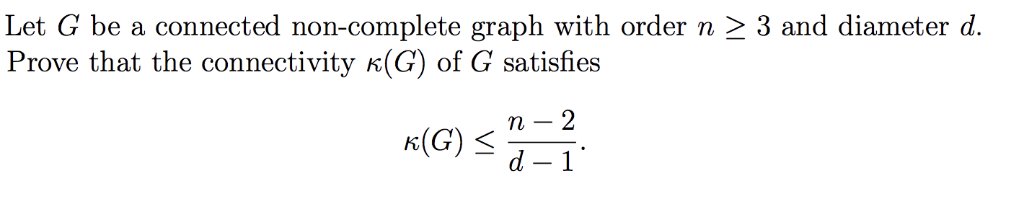Let G be a connected non-complete graph with order n 2 3 and diameter d. Prove that the connectivity κ(G) of G satisfies d-1 Let G be a connected non-complete graph with order n 2 3 and diameter d. Prove that the connectivity κ(G) of G satisfies d-1

• ### 2. (a) Let G be a connected non-complete graph with order n 2 3 and diameter d. Prove that the connectivity K(G) of G satisfies d-1 (b) A connected graph is called unicyclic if it contains exactl...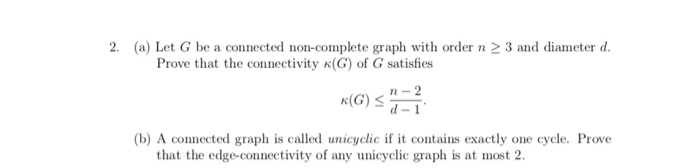2. (a) Let G be a connected non-complete graph with order n 2 3 and diameter d. Prove that the connectivity K(G) of G satisfies d-1 (b) A connected graph is called unicyclic if it contains exactly one cycle. Prove that the edge-connectivity of any unicyclic graph is at most 2. 2. (a) Let G be a connected non-complete graph with order n 2 3 and diameter d. Prove that the connectivity K(G) of G satisfies d-1 (b) A connected...

• ### Prove that the number of vertices of odd degree in a given graph is even.

Prove that the number of vertices of odd degree in a given graph is even.

• ### Let G (V, E) be a directed graph with n vertices and m edges. It is known that in dfsTrace of G t...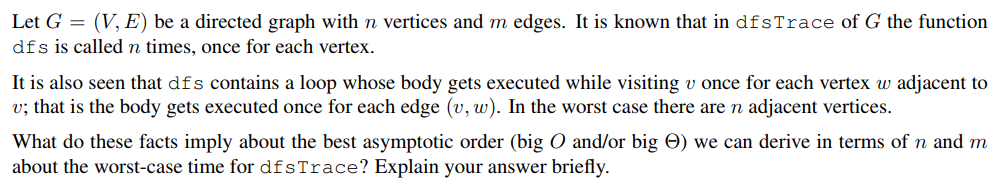Let G (V, E) be a directed graph with n vertices and m edges. It is known that in dfsTrace of G the function dfs is called n times, once for each vertex It is also seen that dfs contains a loop whose body gets executed while visiting v once for each vertex w adjacent to v; that is the body gets executed once for each edge (v, w). In the worst case there are n adjacent vertices. What do...

• ### Prove that, if G is a nontrivial connected graph in which every vertex has even degree, then the ...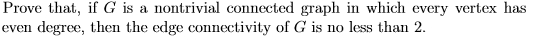Prove that, if G is a nontrivial connected graph in which every vertex has even degree, then the edge connectivity of G is no less than 2. Prove that, if G is a nontrivial connected graph in which every vertex has even degree, then the edge connectivity of G is no less than 2.

• ### Prove that if "T" is a simple graph with "p" vertices, where each vertex has Degree = (p-1)/2,...

Prove that if "T" is a simple graph with "p" vertices, where each vertex has Degree = (p-1)/2, then G must be connected. You may wish to examine the number of verticesin each connected component.

• ### 5. Let G be a graph with order n and size m. Suppose that n 2 3 and n-n2)+2 m > Using Ore's Theorem, prove that G is Hamiltonian 5. Let G be a graph with order n and size m. Suppose t...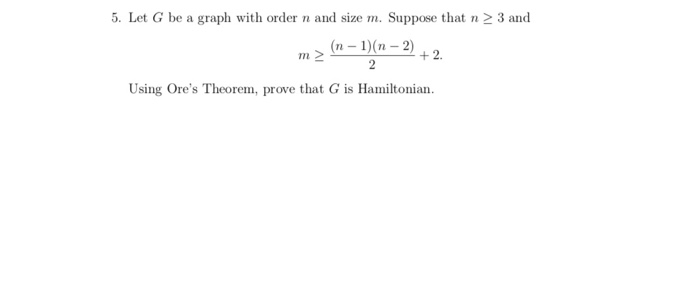5. Let G be a graph with order n and size m. Suppose that n 2 3 and n-n2)+2 m > Using Ore's Theorem, prove that G is Hamiltonian 5. Let G be a graph with order n and size m. Suppose that n 2 3 and n-n2)+2 m > Using Ore's Theorem, prove that G is Hamiltonian

Need Online Homework Help?